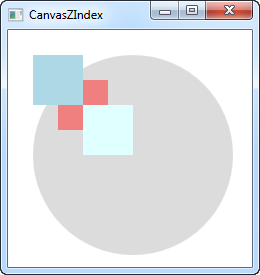# Canvas控件

Canvas（画布）可能是所有面板中最简单的。实际上，它不会默认做任何事，而是允许你把控件放在它的内部，然后显式地给这些控件定位。

``````<Window x:Class="WpfTutorialSamples.Panels.Canvas"
xmlns="http://schemas.microsoft.com/winfx/2006/xaml/presentation"
xmlns:x="http://schemas.microsoft.com/winfx/2006/xaml"
Title="Canvas" Height="200" Width="200">
<Canvas>
<Button>Button 1</Button>
<Button>Button 2</Button>
</Canvas>
</Window>````````````<Window x:Class="WpfTutorialSamples.Panels.Canvas"
xmlns="http://schemas.microsoft.com/winfx/2006/xaml/presentation"
xmlns:x="http://schemas.microsoft.com/winfx/2006/xaml"
Title="Canvas" Height="200" Width="200">
<Canvas>
<Button Canvas.Left="10">Top left</Button>
<Button Canvas.Right="10">Top right</Button>
<Button Canvas.Left="10" Canvas.Bottom="10">Bottom left</Button>
<Button Canvas.Right="10" Canvas.Bottom="10">Bottom right</Button>
</Canvas>
</Window>``````## Z轴

``````<Window x:Class="WpfTutorialSamples.Panels.CanvasZIndex"
xmlns="http://schemas.microsoft.com/winfx/2006/xaml/presentation"
xmlns:x="http://schemas.microsoft.com/winfx/2006/xaml"
Title="CanvasZIndex" Height="275" Width="260">
<Canvas>
<Ellipse Fill="Gainsboro" Canvas.Left="25" Canvas.Top="25" Width="200" Height="200" />
<Rectangle Fill="LightBlue" Canvas.Left="25" Canvas.Top="25" Width="50" Height="50" />
<Rectangle Fill="LightCoral" Canvas.Left="50" Canvas.Top="50" Width="50" Height="50" />
<Rectangle Fill="LightCyan" Canvas.Left="75" Canvas.Top="75" Width="50" Height="50" />
</Canvas>
</Window>````````````<Window x:Class="WpfTutorialSamples.Panels.CanvasZIndex"
xmlns="http://schemas.microsoft.com/winfx/2006/xaml/presentation"
xmlns:x="http://schemas.microsoft.com/winfx/2006/xaml"
Title="CanvasZIndex" Height="275" Width="260">
<Canvas>
<Ellipse Panel.ZIndex="2" Fill="Gainsboro" Canvas.Left="25" Canvas.Top="25" Width="200" Height="200" />
<Rectangle Panel.ZIndex="3" Fill="LightBlue" Canvas.Left="25" Canvas.Top="25" Width="50" Height="50" />
<Rectangle Panel.ZIndex="2" Fill="LightCoral" Canvas.Left="50" Canvas.Top="50" Width="50" Height="50" />
<Rectangle Panel.ZIndex="4" Fill="LightCyan" Canvas.Left="75" Canvas.Top="75" Width="50" Height="50" />
</Canvas>
</Window>``````